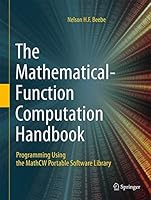# The Mathematical-Function Computation Handbook0 Reviews
2017-09-19
1115 pages

## Book Description

This highly comprehensive handbook provides a substantial advance in the computation of elementary and special functions of , extending the function coverage of major languages well beyond their international standards, including full support for decimal floating-point arithmetic. Written with clarity and focusing on the C language, the work pays extensive attention to little-understood aspects of floating-point and integer arithmetic, and to portability, as well as to important historical architectures. It extends support to a future 256-bit, floating-point format offering 70 decimal digits of precision.

Select Topics and Features: references an exceptionally useful, author-maintained MathCW website, containing source code for the book’s software, compiled libraries for numerous systems, pre-built

C compilers, and other related materials; offers a unique approach to covering mathematical-function computation using decimal arithmetic; provides extremely versatile appendices for interfaces to numerous other languages:  Ada, C#, C++, Fortran, Java, and Pascal; presupposes only basic familiarity with computer programming in a common language, as well as early level ; supplies a library that readily adapts for existing scripting languages, with minimal effort; supports both binary and decimal arithmetic, in up to 10 different floating-point formats; covers a significant portion (with highly accurate implementations) of the U.S National Institute of Standards and Technology’s 10-year to codify mathematical functions.

This highly practical text/reference is an invaluable tool for advanced undergraduates, recording many lessons of the intermingled history of computer hardw

are and software, numerical algorithms, and mathematics. In addition, professional numerical analysts and others will find the handbook of real interest and utility because it builds on research by the mathematical software community over the last four decades.

Chapter 1 Introduction
Chapter 2 Iterative Solutions And Other Tools
Chapter 3 Polynomial Approximations
Chapter 4 Implementation Issues
Chapter 5 The Floating-Point Environment
Chapter 6 Converting Floating-Point To Integers
Chapter 7 Random Numbers
Chapter 8 Roots
Chapter 9 Argument Reduction
Chapter 10 Exponential And Logarithm
Chapter 11 Trigonometric Functions
Chapter 12 Hyperbolic Functions
Chapter 13 Pair-Precision Arithmetic
Chapter 14 Power Function
Chapter 15 Complex Arithmetic Primitives
Chapter 17 Elementary Functions In Complex Arithmetic
Chapter 18 The Greek Functions: Gamma, Psi, And Zeta
Chapter 19 Error And Probability Functions
Chapter 20 Elliptic Integral Functions
Chapter 21 Bessel Functions
Chapter 22 Testing The Library
Chapter 23 Pair-Precision Elementary Functions
Chapter 24 Accuracy Of The Cody/Waite Algorithms
Chapter 25 Improving Upon The Cody/Waite Algorithms
Chapter 26 Floating-Point Output
Chapter 27 Floating-Point Input

Appendix B C# Interface
Appendix C C++ Interface
Appendix D decimal Arithmetic
Appendix E Errata In The Cody/Waite Book
Appendix F Fortran Interface
Appendix H historical Floating-Point Architectures
Appendix I Integer Arithmetic
Appendix J Java Interface
Appendix L Letter Notation
Appendix P Pascal Interface

## Book Details

• Title: The Mathematical-Function Computation Handbook
• Author:
• Length: 1115 pages
• Edition: 1st ed. 2017
• Language: English
• Publisher:
• Publication Date: 2017-09-19
• ISBN-10: 3319641093
• ISBN-13: 9783319641096